# 3 children

3 children had to divide 4 pounds is candy. How much candy did each child get?

x =  1.3333

### Step-by-step explanation:

$x=4\mathrm{/}3=\frac{4}{3}=1\frac{1}{3}=1.3333$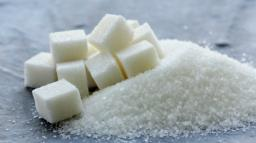Did you find an error or inaccuracy? Feel free to write us. Thank you!Tips to related online calculators
Need help to calculate sum, simplify or multiply fractions? Try our fraction calculator.

## Related math problems and questions:

• PoundsThree pounds subtract 1/3 of a pound.
• Split between 4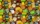There are 12 1/4 pounds of candy canes to split between four classes evenly. How many pounds of the candy canes will each class get?
• LarryLarry received 1/3 pound of candy from his grandmother and 2/5 pound of candy from his best friend. Larry's sister ate 1/2 pound of Larry's candy. How many pounds of candy does Larry have left?
• Kilo candyOne kilogram of chocolate candy cost 10 euros. Kate buys 250 grams and had the 8 pack of candy. How much does cost one bonbon?
• ChocolateChildren break chocolate first to third and then every part of another half. What kind got each child? Draw a picture. What part would have received if each piece have halved?
• School trip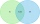On a school trip, 17 of the 28 children bought ice cream or chocolate in a candy store. Twelve children bought chocolate, and nine children bought ice cream. How many children bought ice cream and chocolate? How many children did not buy ice cream? How ma
• Children in the classroom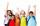How many children are in the classroom, where Ladislav is 10th lightest and 16th heaviest in class?
• Evaluate 11Multiply the quotient of 6 and 2 by 3, then add 1. Add 1 and 6, then divide by 2 and multiply by 3. Divide 6 by the product of 2 and 3, then add 1.
• Lesson exercising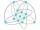The lesson of physical education, pupils are first divided into three groups so that each has the same number. The they redistributed, but into six groups. And again, it was the same number of children in each group. Finally they divided into nine equal g
• PlumsIn the bowl are plums. How many would be there if we can divide it equally among 8, 10 and 11 children?
• On the tripOn the trip were more than 55 children, but less than 65 children. Groups of 7 could be formed, but groups of 8 no. How many children were on a trip?
• FractionsSort fractions z1 = (6)/(11); z2 = (10)/(21); z3 = (19)/(22) by its size. Result write as three serial numbers 1,2,3.
• Dividing walnuts into crates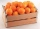There are 8 and 2 over 3 pounds of walnuts in a container, which will be divided equally into containers that hold 1 and 1 over 5 pounds. This would fill n and 4 over 18 containers. What is n?
• GrapesA grocery store sells grapes for \$1.99 per pound. You buy 2.34 pounds of grapes. How much do you pay?
• Trio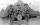56 children lined up in groups of three. How many children did not create a trio?
• Candy barsSheldon has 4 candy bars and wants to split them among his five friends. If each person gets the same amount, what part of the candy bar will each friend get? Show your work.
• Candy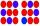How many ways can divide 10 identical candies to 5 children?